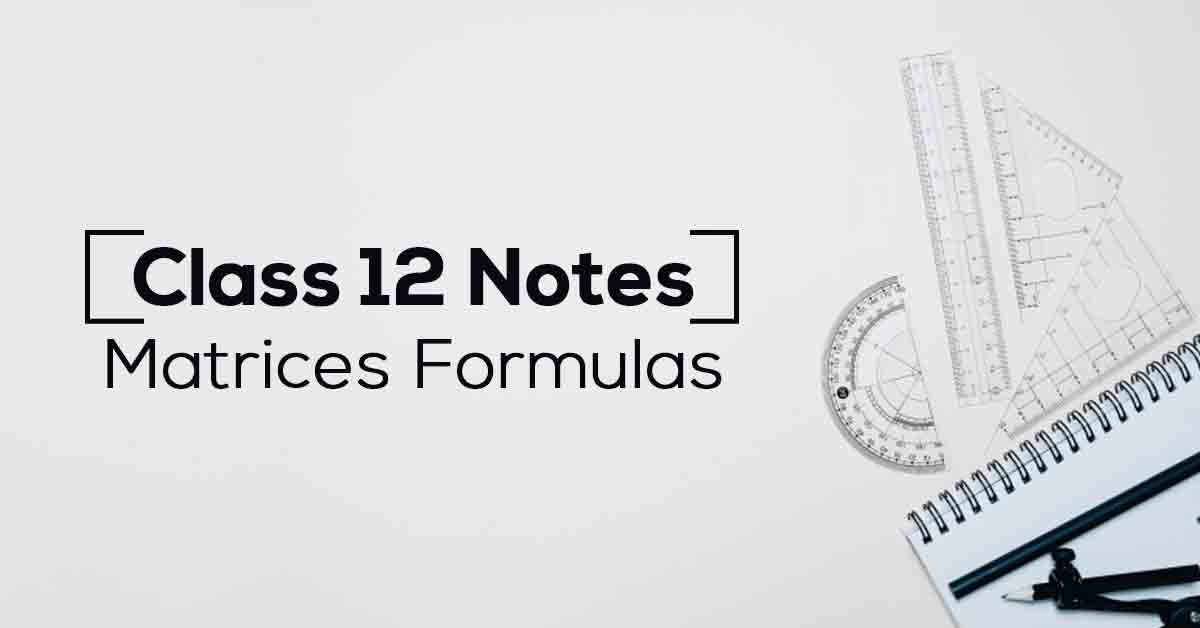Matrices Class 12 Formulas and Notes | Vidyakul
×

# Matrices Class 12 Formulas and Notes## Class 12 Maths Chapter 3 Matrices Class 12 Formulas & Notes – PDF Download

A matrix is a two-dimensional array of numbers, having a fixed number of rows and columns, and containing a number at the intersection of each row and each column. A matrix is usually delimited by square brackets. Know More about these in Matrices Class 12 Formulas and Notes.

The topics and sub-topics covered in Matrices Class 12 Formulas and Notes are:

3.1 Introduction

3.2 Matrix

3.2.1 Order of a matrix

3.3 Types of Matrices

3.3.1 Equality of matrices

3.4 Operations on Matrices

3.4.2 Multiplication of a matrix by a scalar

3.4.4 Properties of scalar multiplication of a matrix

3.4.5 Multiplication of matrices

3.4.6 Properties of multiplication of matrices

3.5. Transpose of a Matrix

3.5.1 Properties of transpose of the matrices

3.6 Symmetric and Skew-Symmetric Matrices

3.7 Elementary Operation (Transformation) of a Matrix

3.8 Invertible Matrices

3.8.1 Inverse of a matrix by elementary operations.

Download the FREE PDF of Matrices Class 12 Formulas and Notes and start your preparation with Vidyakul!